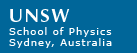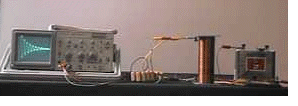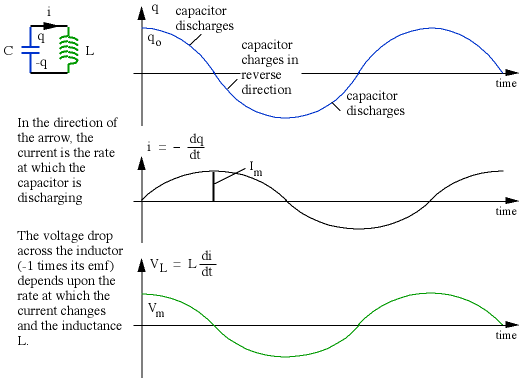# LC Oscillations and Resonance

This page discusses resonance in LC circuits (circuits containing a capacitor and an inductor). For terminology, introductory material and some animations, see AC circuits.A charged capacitor is connected to a non-ideal coil
and the voltage recorded on an oscilloscope.
To begin, let's look at a circuit that has only a capacitor and an inductor. We shall pretend that these components are ideal, ie that they have no internal resistance. In practice this is not possible: there will always be some way in which electrical energy can be converted to heat, even if the rate is very small. We begin by charging the capacitor C with an initial charge qo. (In the demonstration shown in the photograph above, we did this by connecting the capacitor to a battery, then disconnecting it.) We then connect the capacitor to an inductance L, as shown below, and analyse the current i that flows and the voltage V across the components.We can substitute this solution back into the differential equation to check that it works. Now let's look at that solution.Provided that the components are ideal, as assumed, the charge flows back and forwards for ever, with angular frequency wo, or with cyclic frequency f = w/2p. The energy comes from the fact that we initially charged the capacitor with an energy (1/2)qo2/C, which gives it a potential difference V = qo/C. This potential difference initially drives the charge off the capacitor. The obvious question is why the system doesn't cease changing when the capacitor is discharged. The answer is that by this time there is a current flowing through the inductor, and one cannot rapidly change the current in an inductor because that would produce a large emf. In fact the energy at this stage is stored in the magnetic field of the inductor, whose potential energy is (1/2)L.im2. The current and the voltage for both components are out of phase by 90° (see AC circuits), and so the energy is transformed from electrical to magnetic and back again, as shown below.One can make an analogy with the simple harmonic motion of a pendulum, whose energy is transformed from gravitational potential energy to kinetic energy and back again. In the case of the LC circuit, the potential energy in the inductor could be considered analogous to kinetic energy, because it depends upon the current, ie the motion of the charge. When that charge starts to "pile up" on the capacitor, it produces the electrical potential energy (cf gravitational potential energy) which tends to force it back. Eventually it reaches maximum charge (cf maximum height) and starts to discharge, with the current increasing in the opposite direction. This is only an analogy: the momentum of the electrons themselves is negligible, and it is the magnetic field in the inductor that keeps them moving.

The algebra refers to ideal components, which are hard to find in practice. Inductors almost always have internal resistance. Consequently some electrical energy is lost as heat in this or other resistances, and there is also some loss due to electromagnetic radiation. Each cycle loses a little energy, so the oscillations in practice fall gradually to zero, as shown in the photograph at the top of this page.

Now go back to the resonance section of AC circuits.

Joe Wolfe / J.Wolfe@unsw.edu.au, phone 61- 2-9385 4954 (UT + 10, +11 Oct-Mar).

School of Physics, University of New South Wales, Sydney, Australia.

There are pages on related material atThis work is licensed under a Creative Commons License.